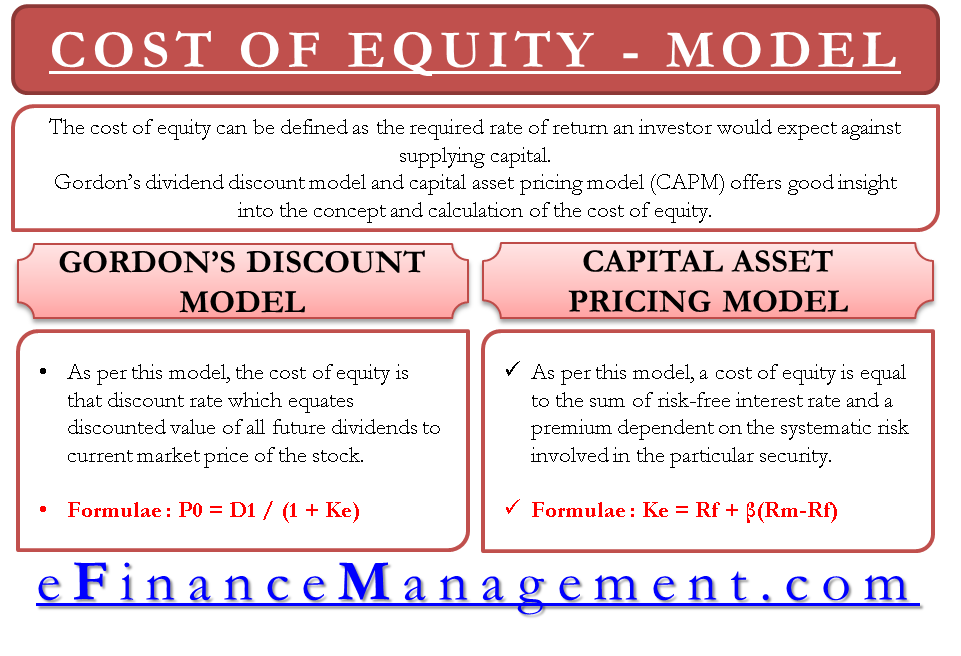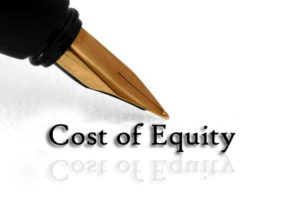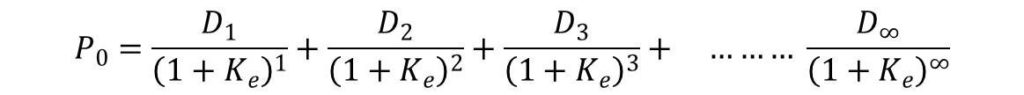# Models for Calculating Cost of Equity

The cost of equity can be defined as the required rate of return an investor would expect against supplying capital. The Expected rate of return has a direct relation with risk. Higher the risk, higher would be the expected returns. Gordon’s dividend discount model and capital asset pricing model (CAPM) offers good insight into the concept and calculation of the cost of equity.

The cost of any security is the return that a company pays for using the capital. Like interest cost, at a specified percentage, is the cost of debt capital. Similarly, dividend, at a specific rate, paid to preference shareholders is the cost of preference dividend. If we think in similar fashion, what is the return to equity shareholders? Return is in the form of dividends. But, dividend payment by any company is not fixed at a specified rate. It is not even mandatory by law unlike other securities – debt and preference shares.## Models For Calculating Cost of Equity

Fundamentally, there is no direct measure of the cost of equity. In the absence of that, it is very difficult to measure the cost of equity. There are variousmodels to help us in finding the cost of equity based on various assumptions. Let’s look at the most prominent models for estimating the cost of equity.

## Gordon’s Dividend Discount Model

This model is also known as discount capitalization model. As per this model, the cost of equity is that discount rate which equates discounted value of all the future dividends to the current market price of the stock. It assumes that present value of all the future dividends is reflected in the market price of the stock. In the form of formula/ equation, it is represented as below:Cost of Equity Formula

In the above equation, P0 is the current market price, D is the dividend year wise and Ke is the cost of equity.

The equation will be simplified if the growth of dividend is constant. Let us suppose the growth to be ‘g’.

## Capital Asset Pricing Model (CAPM)

This model is a very well-known model and is widely used in the industry. As per this model, a cost of equity is equal to the sum of risk-free interest rate and a premium dependent on the systematic risk involved in the particular security. This model directly focuses on the relationship between the risk and required return. The views of CAPM are expressed by the following formula/ equation:

Cost of Equity = Rf + β*(Rm-Rf)

Here,  is the cost of equity,  is the risk-free rate,  is the expected return for the market portfolio, and is beta.

Beta (β):  Beta is the representative of systematic risk in the equation. Systematic risk is that risk which is not avoidable by diversification.

Expected Return of the Market Portfolio (Rm):  It is the average return of all the securities of the market. Some market securities pay higher and some other lower than the expected return of the market portfolio.

Here, the second part of the equation is the deciding factor. The cost of equity depends on the beta. If the beta is 1, the security’s required rate of return would be equal to Rm. In case, the beta is less than 1,  would be lower than Rm and vice versa.

Last updated on : May 13th, 2019
What’s your view on this? Share it in comments below.